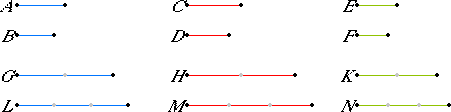# Proposition 11

Ratios which are the same with the same ratio are also the same with one another.

Let A be to B as C is to D, and let C be to D as E is to F.

I say that A is to B as E is to F.

Take equimultiples G, H, and K of A, C, and E, and take other, arbitrary, equimultiples L, M, and N of B, D, and F.V.Def.5

Then since A is to B as C is to D, and of A and C equimultiples G and H have been taken, and of B and D other, arbitrary, equimultiples L and M, therefore, if G is in excess of L, H is also in excess of M; if equal, equal; and if less, less.

Again, since C is to D as E is to F, and of C and E equimultiples H and K have been taken, and of D and F other, arbitrary, equimultiples M and N, therefore, if H is in excess of M, K is also in excess of N; if equal, equal; and if less, less.

V.Def.5

But we saw that, if H was in excess of M, G was also in excess of L; if equal, equal; and if less, less, so that, in addition, if G is in excess of L, K is also in excess of N; if equal, equal; and if less, less.

V.Def.5

And G and K are equimultiples of A and E, while L and N are other, arbitrary, equimultiples of B and F, therefore A is to B as E is to F.

Therefore, ratios which are the same with the same ratio are also the same with one another.

Q.E.D.

## Guide

This proposition expresses the transitivity of the relation of being the same when applied to ratios. After this proposition (and the easily proved properties of reflexivity and symmetry, see the Guide to definition V.Def.6), the expression “two ratios are the same,” or the equivalent expression “two ratios are equal,” is justified. The proof follows directly from the definition. What is remarkable is that Eudoxus, or Euclid, recognized that this proposition needed to be proved.

The magnitudes may be of three different kinds with A and B of one kind, C and D of a second kind, and E and F of a third kind.

This proposition is used very frequently whenever ratios are used.